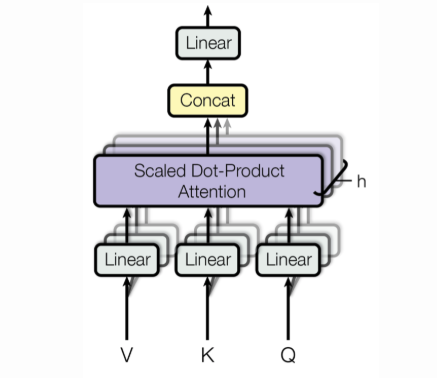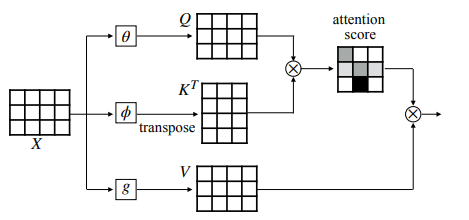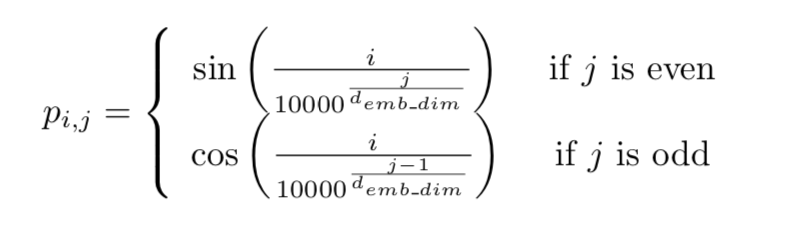## Oracle AI & Data Science BlogLearn AI, ML, and data science best practicesIn this blog, we will be discussing recent research done by the Google Team bringing state-of-the-art results in the area of natural language processing.

Till now, we have widely been using LSTMs and GRUs for sequential data, as they seem to capture better positional and semantic information. Despite the popularity, the drawback of using them is a high computational requirement and complex architecture. So, what to do then?

Google's research paper "Attention Is All You Need" proposes an alternative way for using recurrent neural networks (RNNs) and still getting better results. They have introduced a concept of transformers which is based on Multi-Head Self-Attention; we will be discussing more about the term here.

Subscribe to the Oracle AI & Data Science Blog to get the latest posts sent straight to your inbox!

## Self-Attention

We know that attention is a mechanism to find the words of importance for a given query word in a sentence. The mathematical representation for the attention mechanism looks like the figure given below:So, X is the input word sequence, and we calculate three values from that which is Q(Query)K(Key) and V(Value).

The task is to find the important words from the Keys for the Query word. This is done by passing the query and key to a mathematical function (usually matrix multiplication followed by softmax). The resulting context vector for Q is the multiplication of the probability vector obtained by the softmax with the Value.

When the Query, Key, and Value are all generated from the same input sequence X, it is called Self-Attention.We have been breaking into the concept word by word so far, and the only new term here is Multi-Head now. This is just doing the same thing discussed earlier by different heads (brains?). The aim here is to combine the knowledge explored by multiple heads or agents instead of doing it by one, as in the traditional case.

Mathematically, it relates to attending to not only the different words of the sentence, but to different segments of the words, too. The words vectors are divided into a fixed number (h, number of heads) of chunks, and then self-attention is applied on the corresponding chunks, resulting in h context vector for each word. The final context vector is obtained by concatenating all those h context vectors.The figure above is a visualization of the outputs upon using two heads. We can see that if the Query word is it, the first head focuses more on the words the animal, and the second head focuses more on the word tired. Hence, the final context representation will be focusing on all the words the, animal and tired, and thus is a superior representation as compared to the traditional way.

## Positional Embeddings

Now that we have understood self-attention and multi-head self-attention, we can recall the claim that they can be a way to replace RNNs in a language modelling problem.

The question is, HOW?

This is exactly where we should discuss about the positional embeddings. They are nothing but a way to capture the positional information of words in a sentence, as the usual word2vec or Glove embeddings contains only the semantic meanings and do not tell anything about the positions of the words.

We can use a simple one-hot vector and do some kind of mathematical computation using the positional one-hot vectors and the word-embeddings to obtain the positional embeddings. But that would be a very naive approach.

Google uses the sin and cosine functions to compute the position vectors, as it normalises the position between -1 to 1 and thus is a good scaling solution for long sequences. The mathematical formula for computing the same looks as follows:Once we have calculated the positional encodings, we can combine them with the respective word embeddings to get the final positional word embeddings.Hence, the conclusion is that attention alone can work sufficiently as the replacement for the RNN-based architectures and, besides that, using them is quite faster and even produces better results.

AMAZING, isn’t it?

Hope you liked the explanation. Keep Learning, Keep Sharing :)